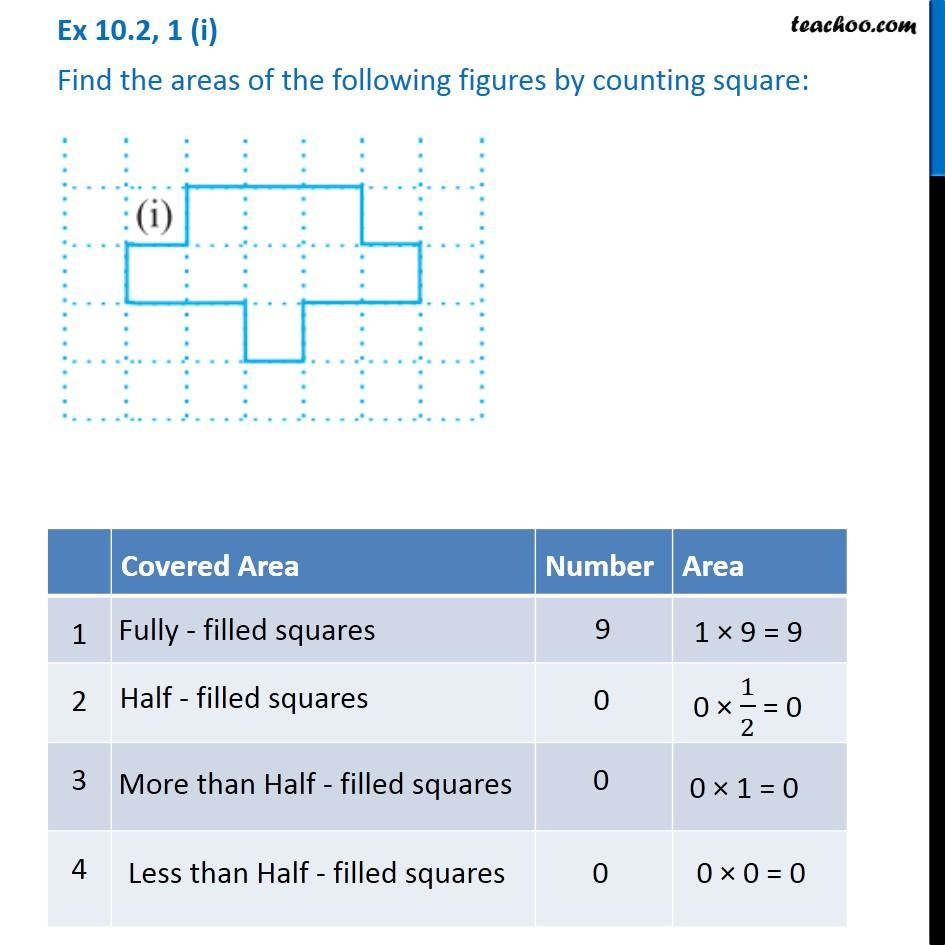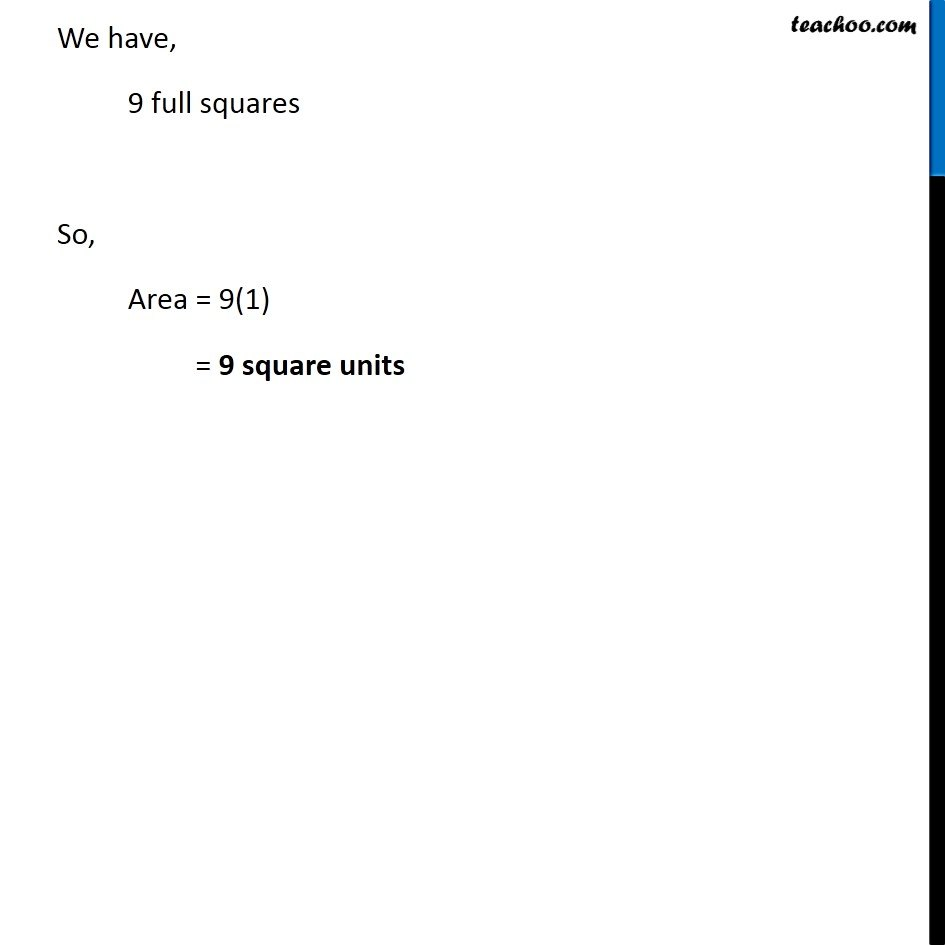1. Chapter 10 Class 6 Mensuration
2. Concept wise
3. Finding area by counting squares

Transcript

Ex 10.2, 1 (i) Find the areas of the following figures by counting square: Covered Area Number Area 1 Fully filled squares 9 1 × 9 = 9 2 Half filled squares 0 0 × 1/2 = 0 3 More than Half filled squares 0 0 × 1 = 0 4 Less than half filled squares 0 0 × 0 = 0. We have, 9 full squares So, Area = 9(1) = 9 square units